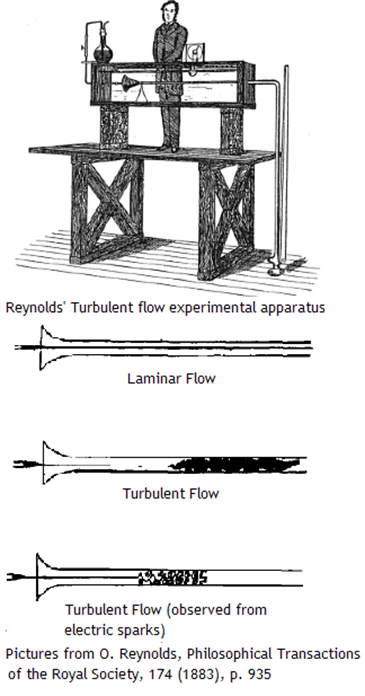# Reynolds number and dynamic similarity of fluid flows

Previous pageWhen analyzing a fluid flow,  first of all fluid dynamicists calculate the Reynolds number, because its value tells them a lot.

Reynolds number is named after Osborne Reynolds, who was the first to notice that the character of the flow is determined by this number. Reynolds number is usually denoted Re.

If two flows past similar bodies  have the same Reynolds number, they are dynamically similar. Dynamic similarity has several uses.

• Solving the non-dimensional Navier-Stokes equations for one Re produces the solution for an infinite number of real flows past geometrically similar bodies all having the same Re. This is why Flow Illustrator does not require the user to provide the actual dimensions of the object, the actual value of the velocity far upstream, and the properties of the fluid: Re is enough.
• Wind-tunnel experiments do not have to be performed at the same velocity and other conditions as in the flight regime: it is sufficient to have the same Re, and then to rescale the results.
• If the velocity and length scales used are relevant, the Reynolds number has the physical meaning of the ratio of inertial forces to viscous forces. Then the nature of the flow can be judged by the value of the Reynolds number alone rather than by the combination of velocity, size, and fluid properties.

This is why experienced fluid dynamicists calculate the Reynolds number using the relevant scales. If thus calculated Re is very small, say, less than 1, even for bluff bodies like a circular cylinder the flow will remain attached, and will become steady after the initial transient. In Flow Illustrator this can be observed by the vorticity distribution becoming time-independent after the transient. As Re increases, the flow becomes unsteady, at first with time-periodic vortex shedding, and then loosing periodicity and eventually becoming turbulent. Also, with large Re thin boundary layers form near the surface. This, too, can be observed in Flow Illustrator by the behavior of the vorticity.

What exactly is Re and what exactly is dynamic similarity? Two geometric shapes are called similar if they can be made identical by scaling. Two flows are also called similar if they can be made identical by scaling. In case of fluid flows scaling can be applied both to the coordinates and to the flow parameters like velocity and pressure.

Consider, for example, two flows past geometrically similar aerofoils with the same angle of attack. Each flow has its own value of velocity far upstream, and the physical properties of the fluid are also different: say, in one case the fluid is air, and in another case the fluid is water.

Now, let us do rescaling. As a unit of length we will use the chord length of each aerofoil. The result is that in the new units the chord length of each aerofoil becomes exactly 1, and it is now non-dimensional. Since the shapes are similar, the aerofoils will also have the same non-dimensional maximum thickness, and all other geometrical characteristics will also be identical.

As a unit of velocity, we will use the velocity far upstream. Then, in these new units in both flows the velocity far upstream will be equal to one, that is it will be the same.

Now, an interesting question is: in these non-dimensional units will the velocities of these two flows be identical not only far upstream but everywhere?

In general, the answer is no. One can rewrite the NavierStokes equations governing the fluid motion in these new rescaled variables. It turns out that they will be almost identical, that is identical with an exception of one coefficient only, which is equal to the product of the length scale and the velocity scale, divided by kinematic viscosity. This coefficient is called Reynolds number. If the velocity scale is U, the length scale is L, and the kinematic viscosity is ν, then the Reynolds number Re=UL/ν. If our two flows are characterised by different values of Re, then the solutions will be different.

However, if it happens that these two flows have not only similar geometry (and, actually, similar boundary conditions, like the angle of attack for example), but also the same Re, then in non-dimensional form the entire flows will be identical. Such flows are called dynamically similar.